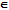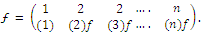# Describe the symmetric set of degree n

Publish On: 2019-03-18

Total Post: 559

# Mjay Jollay

Total Post: 0

## ANS: Describe the symmetric set of degree n

If S is a finite set containing n distinct elements, then there will be n! distinct arrangements of the elements of set S. The set of all these n! arrangements is denoted by Sn and is known as symmetric set of permutations of degree n or symmetric set of degree n. Also

Sn = {ƒ : ƒ is a permutation of degree n}

Obviously, a set of three elements will have 3! Elements, i.e. S3 will have been 3! Or 6 elements.

Let S = {1, 2, 3, …., n}.

Let ƒSn be a permutation of Sn. This can be exhibited asSince ƒ is one-one onto, so we have

n choices for (1)ƒ

(n – 1) choices for (2)ƒ

………….

………….

1 choice for (n)ƒ

Hence in all n(n – 1) (n – 2) …… 3.2.1. = n! functions ƒSn can arise. Thus Sn has n! elements.